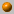##### Personal tools
You are here: Home Computational Geometry - Spring 2010
« February 2023 »
February
SuMoTuWeThFrSa
1234
567891011
12131415161718
19202122232425
262728

# Computational Geometry - Spring 2010

Instructor: Dan Halperin , danha AT post

Monday, 16:00--19:00

Office hours: Monday, 19:00--20:00
Kaplun 118

The course covers fundamental algorithms for solving geometric problems such as computing convex hulls, intersection of line segments, Voronoi diagrams, polygon triangulation, and linear programming in low dimensional space. We will also discuss several applications of geometric algorithms to solving problems in robotics, GIS (geographic information systems), computer graphics, and more.

### Bibliography:

The main textbook of the course is
Computational Geometry: Algorithms and Applications (CGAA), 2nd or 3rd edition
by M. de Berg, M. van Kreveld, M. Overmars, and O. Schwarzkopf.

A bibliographic list for the course

The assignments, which will be graded by the students, will account for 25% of the final grade, in case they improve the final grade. The grade in the final exam, to be held on Monday, June 28th, 2010, will account for the remaning 75% of the grade in the course.

Although the assignment grades will be used only for improving the final grade, submission of the assignments is mandatory and a prerequisite for taking the exam.

Assignment no. 1 (pdf file), due: March 15th, 2010.

Assignment no. 2 (pdf file), due: April 12th, 2010.

Assignment no. 3 (pdf file), due: May 24th, 2010.

Assignment no. 4 (pdf file), due: June 7th, 2010.

### Course Summary:

Below you'll find a very brief summary of what was covered in class during the semester. This should not be taken as a complete description of the course's contents.

For an outline of the course, see for example, the course summary in the 2008 computational geometry course .22.2.10
What is Computational Geometry? Course outline, motivation and techniques (to be continued). Naive convex hull algorithms (see Chapter 3 in O'Rourke's book). Orientation (Side-of-line) test. Gift wrapping. Graham's O(n log n) algorithm (Chapter 1 in CGAA).1.3.10
Convex hull computation continued; Line segment intersection

Graham's O(n log n) algorithm (Chapter 1 in CGAA). Lower bound for convex hull algorithms.

Output sensitive algorithm to compute the intersections of line segments: Bentley Ottmann's plane sweep (Chapter 2 in CGAA).8.3.10
The doubly-connected edge list (DCEL), and overlay of planar subdivisions (CGAA, Chapter 2).

A proof of Euler's formula (from "Proofs from The Book" by Aigner and Ziegler).
http://en.wikipedia.org/wiki/Ramer-Douglas-Peucker_algorithm.
whose References include the Hershberger-Snoeyink improvement that we disucssed).15.3.10

Polygon triangulation; Art gallery problems

We have shown that \$\lfloor n/3 \rfloor\$ guards are sometimes necessary and always sufficient to guard a simple polygon with n vertices. We have shown that every simple polygon can be triangulated; that the triangulation graph is 3-colorable; that the dual of the triangulation graph is a tree.

A naive algorithm that mimics the triangulation existence proof can triangulate a simple polygon with n vertices in O(n^3) time. A more efficient algorithm does it in O(n\log n) by first decomposing a polygon into monotone polygons and then triangulating each of the resulting monotone subpolygons.

The material covered in class could be found in O'Rourke's book, Chapters 1 and 2, and in CGAA, Chapter 3. (The description of how to decompose a polygon into y-monotone polygons follows O'Rourke's presentation.)
We have also shown that \Omega(n^{3/2} guards are sometimes needed to guard a polyhedron with n vertices. The construction is due to Seidel and its presentation is taken from the book Art Gallery Theorems and Algorithms by J. O'Rourke.

Finally, getting back to map overlay, we noticed the implication of map overlay to Boolean operations on polygons;
see CGAA Section 2.4.22.03.1012.4.10
The casting problem. Half plane intersection. Introduction to Linear Programming. A deterministic incremental algorithm for LP in 2D (CGAA, Chapter 4).26.4.10
Linear Programming continued. A randomized algorithm for LP in 2D. The unbounded case in 2D;
sketch of LP in 3D. The minimum enclosing disk problem (CGAA, Chapter 4). The in-circle test.3.5.10
More details on the minimum enclosing disk algorithm and the in-circle test.
Orthogonal range search I: kd-trees and range-trees. CGAA, Chapter 5.10.5.10
This lesson will last only till 17:10. A make-up lesson will be held on Friday, May 28th, at 10:00 (more details will be given closer to this day).
Orthogonal range search II: The high-dimensional case. Relaxing the general position assumption. Fractional cascading. CGAA, Chapter 5.17.5.10
Trapezoidal maps and planar point location. CGAA, Chapter 6.24.5.10
Two guest talks: Raimund Seidel from the University of Saarland on Barckward Analysis of Randomized Algorithms, and Pankaj Agarwal from Duke University on Core-Sets.
Introduction to Voronoi diagrams.28.5.10 Friday (make-up lesson)
Voronoi diagrams of points in the plane: structure, complexity, anf Fortune's algorithm. CGAA, Chapter 7.31.5.10
Point-Line duality, arrangements of lines in the plane, the zone theorem and incremental construction of the arrangement. Application to computing the half-plane discrepancy in a square. CGAA, Chapter 8. We also showed an application to finding the minimum area triangle determined by a set of points in the plane; see the paper The Power of Geometric Duality, by Chazelle et al.

##### Document Actions